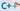# Quick C++ Input Stream Explanation

February 26, 2018

The issue is with the way the different c++ input stream readers/extractors work internally. Basically the various input stream operators/functions (>>, getchar, getline, etc) internally move a pointer along the input source to indicate where they last stopped reading.

So let us use ^ to denote a pointer to where any of our input stream readers stopped reading in an input stream.

We would also be using the following input to explain how each one works.

``2\n``
``^``
``1 2 3\n``
``6 7 8\n``

The ‘\n’ is used to denote the character representing a newline. You would also notice that I placed a ^ to indicate the initial position of the input reader.

## 1. >>

The >> is a binary operator that accepts an input stream and a variable to store the location of the next input. You can check here for the different overloaded definitions for it with respect to an input stream.

So if it is used in an expression such as this:

``cin >> x;``

It basically says, from the current pointer in the input stream, skip any whitespace or newline which might be there and then keep reading as long as possible & necessary to get a valid representation of x.

So if x in an int it tries to keep reading till it gets a valid integer representation. If x is a double it keeps reading till it gets a valid double representation, and so on and so forth.

So if we define x as an int and we call cin >> x; on our test input from above, the position of the input pointer would be updated to as follows:

``2\n``
`` ^``
``1 2 3\n``
``6 7 8\n``

2 would be stored in x and the pointer would move on to the next line after 2. Now if we call cin >> x; as second time, the input pointer would be updated to as follows:

``2\n``
``1 2 3\n``
`` ^``
``6 7 8\n``

So what happened here…. It skipped the newline it was currently on and then it encountered 1 it would then attempt to parse 1 into an integer, stores it in x and moves the reading pointer to the next character.

## 2. getline()

getline() starts reading all characters from the current position of the input pointer till it encounters a delimiter you specify (the delimiter defaults to a newline if none is specified). So if we call getline(cin, str) for our test input. The position of the input pointer would be updated like this:

``2\n``
``1 2 3\n``
``^``
``6 7 8\n``

Two things to note from here:

1. The pointer does not stop on the new line encountered, instead it is move to the next character in the input.

2. Secondly, the value that will be stored in str will not include that newline character encountered.

So the value in str would be 2 (without the newline). And if we call getline(cin, str) a second time, the value that would be store in str would be 1 2 3 (without the newline) and the pointer would be updated like so:

``2\n``
``1 2 3\n``
``6 7 8\n``
``^``

Now with these in mind, let me address why `getline()` is not working properly for you in that gist (you might probably have guessed it already).

## Solution to the Problem in the Tweet

So you have a sample input of the format:

``2 2``
``3 1 5 4``
``5 1 2 8 9 3``

And you want to read the first two integers and then the next two lines. And you write a code like this:

``int x, y;``
``string line1, line2;``

cin >> x >> y;

getline(cin, line1);

getline(cin, line2);

What would actually be read into the variables are:

``x == 2``
``y == 2``
``line1 == '' // this is not right``
``line2 == '3 1 5 4'``

This happens because after cin reads in the y value, it moves the read pointer to the \n character after the second 2 integer. Now when you call getline() for the first time, it start to reading from the \n, and since it immediately encounters a newline character, it just stores an empty string in line1 and then moves the pointer to the next character (3) after the \n. So the second call to getline() will start reading from 3 till it encounters the next \n character which is after 4 and then store all it has read into line2.

## How to prevent this issue

There are many ways to prevent this from happening, a quick way is to just call getchar() after using >> to read the \n and advance the pointer:

``int x, y;``
``string line1, line2;``
``cin >> x >> y;``
``getchar(); // would read the last \n and advance to next``
``getline(cin, line1);``
``getline(cin, line2);``

Another way is to just use the >> operator to read in all the input that you require. Since you are really after reading in the integers, it is best to allow >> to parse the integers from the input for you like this:

``int x, y;``
``int line1Size;``
``cin >> x >> y;``
``cin >> line1Size;``
``vector<int> line1(line1Size);``
``for(int i = 0; i < line1Size; i++) {``
``  cin >> line1[i];``
``}``
``// repeat for line2``

line1 would then contain the values are required.

Hope this was helpful to understand how to best read inputs in those algorithm contests. Don’t hesitate to comment about your ideas and tricks on reading inputs using c++.